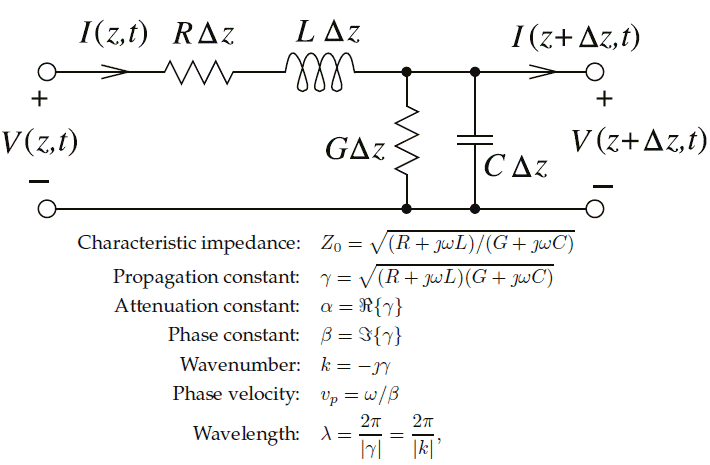# Transmission Line Quantities Calculator

Results:## Online Transmission Line Calculator

An online transmission line calculator is a web-based tool that allows users to calculate various parameters associated with transmission lines. These parameters include:
• Characteristic impedance (Z0): The online calculator can compute the characteristic impedance of a transmission line, which is the ratio of voltage to current in a transmission line under steady-state conditions. It is an important parameter that characterizes the electrical behavior of a transmission line.
• Propagation constant (γ): The propagation constant is a complex-valued parameter that represents the rate of change of voltage and current along a transmission line. It consists of an attenuation constant (alpha) and a phase constant (beta) and is commonly denoted as gamma.
• Attenuation constant (α): The attenuation constant represents the loss of signal power per unit length along a transmission line. It is a real-valued parameter and is typically expressed in Nepers per meter or decibels per meter.
• Phase constant (β): The phase constant represents the phase shift of a signal as it propagates along a transmission line. It is a real-valued parameter and is typically expressed in radians per meter.
• Wavenumber (k): The wavenumber is a measure of the spatial frequency of a signal propagating along a transmission line. It is the magnitude of the phase constant (beta) and is typically expressed in radians per meter.
• Phase velocity (vp): The phase velocity represents the speed at which a signal propagates along a transmission line. It is calculated as the ratio of the wavenumber (k) to the angular frequency (omega) of the signal.
• Wavelength (λ): The wavelength is the distance between two consecutive points in a waveform that are in phase. It is calculated as the ratio of the speed of light in a vacuum to the angular frequency (omega) of the signal.
An online transmission line calculator can be a useful tool for engineers, students, and researchers working with transmission lines, allowing them to quickly and accurately calculate these important parameters for various transmission line configurations and operating frequencies.